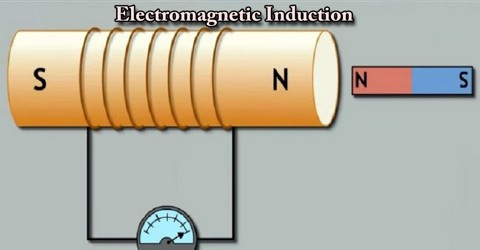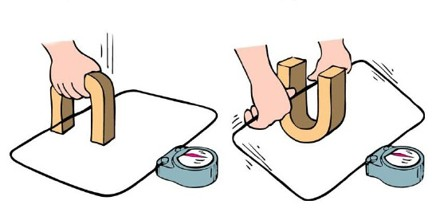Physics

# Electromagnetic InductionElectromagnetic Induction

Definition

Electromagnetic Induction is the production of voltage or electromotive force due to a change in the magnetic field. Electromagnetic induction was discovered by Michael Faraday in the 1830s. Many electrical components and types of equipment work based on the principle of electromagnetic induction. It has found many applications in technology, including electrical components such as inductors and transformers, and devices such as electric motors and generators.Electromagnetic Induction method is based on the measurement of the change in mutual impedance between a pair of coils on or above the earth’s surface. Most Electromagnetic instruments are comprised of two or more sets of coils. These coils are electrically connected and are separated by a fixed distance. The transmitter coil is used to generate an electromagnetic field at a specific frequency. This is known as the primary field. The primary field causes electrical currents to flow in conductive materials in the subsurface. The flow of currents in the subsurface, called eddy currents, generate a secondary magnetic field, which is sensed by the receiver coil. The magnitude of the secondary field sensed by the receiver depends upon the type and distribution of conductive material in the subsurface. Both the induced secondary field, along with the primary field, is detected at the receiver coil. The number of turns in the coils/wire is directly proportional to induced voltage. The induced voltage in an electromagnetic induction is described by the following equation as:

e = N × dΦdt

Where,

e = voltage induced (measured in volts)

t = time (measured in seconds)

N = number of turns found in the coil

Φ = magnetic flux (measured in Webers)

Many types of electrical equipment such as motors, generators and transformers function based on the principle of the electromagnetic induction.Applications of Electromagnetic Induction

Electromagnetic Induction is the production of an electromotive force (i.e., voltage) across an electrical conductor due to its dynamic interaction with a magnetic field. Induction is used in power generation and power transmission, and it’s worth taking a look at how that’s done. There are other effects with some interesting applications to consider, too, such as eddy currents.

An Electric Generator – An electric motor is a device for transforming electrical energy into mechanical energy; an electric generator does the reverse, using mechanical energy to generate electricity. At the heart of both motors and generators is a wire coil in a magnetic field. In fact, the same device can be used as a motor or a generator.

When the device is used as a motor, a current is passed through the coil. The interaction of the magnetic field with the current causes the coil to spin. To use the device as a generator, the coil can be spun, inducing a current in the coil.

Eddy Currents – An eddy current is a swirling current set up in a conductor in response to a changing magnetic field. By Lenzıs law, the current swirls in such a way as to create a magnetic field opposing the change; to do this in a conductor, electrons swirl in a plane perpendicular to the magnetic field. More accurately, eddy currents transform more useful forms of energy, such as kinetic energy, into heat, which is generally much less useful. In many applications the loss of useful energy is not particularly desirable, but there are some practical applications.

Transformers – Electricity is often generated a long way from where it is used, and is transmitted long distances through power lines. Although the resistance of a short length of power line is relatively low, over a long distance the resistance can become substantial. A power line of resistance R causes a power loss of I2R; this is wasted as heat. By reducing the current, therefore, the I2R losses can be minimized. A transformer is made up of two coils, each with a different number of loops, linked by an iron core so the magnetic flux from one passes through the other. When the flux generated by one coil changes, as it does continually if the coil is connected to an AC power source, the flux passing through the other will change, inducing a voltage in the second coil. With AC power, the voltage induced in the second coil will also be AC.